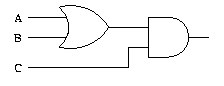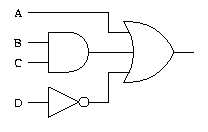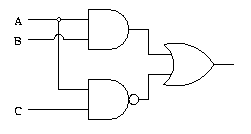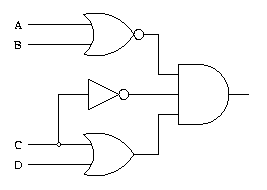Saturday , 24 July 2021
Breaking News

# Basic Laws of Boolean Algebra in Digital Electronics

The basic Laws of Boolean Algebra in Digital Electronics can be specified as follows:

1. Commutative Law conditions that the interchanging of the instruction of operands in a Boolean calculation does not modify its result. For example:
1. OR operator → A + B = B + A
2. AND operator → A * B = B * A
2. The associative Law
1. The associative law of multiplication states that the AND operation are done on two or more than two variables. For example:
A * (B * C) = (A * B) * C
3. Distributive Law
1. Distributive law states that the multiplication of two variables and adding the result with a variable will result in the same value as the multiplication of the addition of the variable with individual variables. For example:
A + BC = (A + B) (A + C).
4. Annulment law:
A.0 = 0
A + 1 = 1
5. Identity law:
A.1 = A
A + 0 = A
6. Idempotent law:
A + A = A
A.A = A
7. Complement law:
A + A’ = 1
A.A’= 0
8. Double negation law:
((A)’)’ = A
9. Absorption law:
A.(A+B) = A
A + AB = A

• Draw a logic circuit for (A + B)C.• Draw a logic circuit for A + BC + D.• Draw a logic circuit for AB + AC.• Draw a logic circuit for (A + B)(C + D)C.